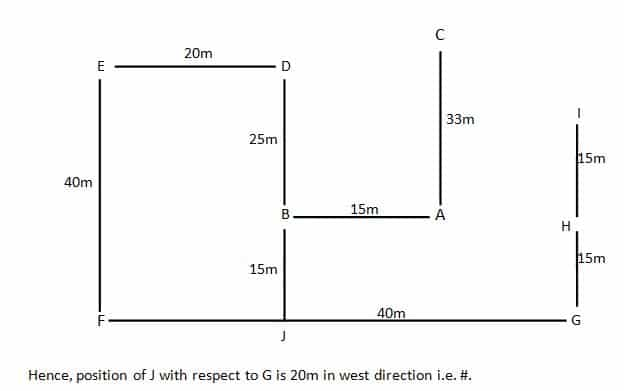# Reasoning Questions : Direction Sense Set 19

Hello Aspirants.

Welcome to Online Reasoning Section in AffairsCloud.com.Here we are creating Best question samples from Direction Sense test with explanation, which is common for IBPS, SBI, RBI, IPPB, LIC, SSC and other  competitive exams. We have included Some questions that are repeatedly asked in bank exams !!!

Direction

Direction (1 – 10): Read the following information carefully and answer the questions given beside.

In the following questions, the symbols &, %, * and # are used with the following meanings as illustrated below. Study the following information and answer the given questions:
Note: The directions which are given indicates exact directions.
A&B – A is in the south direction of B.
A*B – A is in the north direction of B.
A%B – A is in the east direction of B.
A#B – A is in the west direction of B.
A@BS- A is the mid-point of BS vertically.
Note– For southeast direction it used to be written as A&%B and so on…
When it is given that the Car honks once then it will be considered as the car taken a left turn and if it is given as the car honks twice then it will be considered as the car takes a right turn.
Point A is %15m of point B. Point C is *33m of point A. Point D is *25m of point B. Point E is #20m of point D. Point F is &40m of point E. Point G is %40m point F. Point H@IG. Point I is *30m of Point G.

1. What distance the car has to travel from point I to reach the airplane which is parked at point A?
1) 22m
2) 6√10 m
3) 43 m
4) 5√10m
5) 35m
Answer & Explanation
Answer – 4) 5√10m
Solution:Distance between point I and point C = √(152 + 52) = √225 + 25 m = √250 m = 5√10 m
2. What could the possible shortest route to reach point D from point C?
1) Started in east till 15km, honks once, cover 8km
2) Started in west till 15km, honks twice, cover 8km
3) Started in south till 25km, honks once, cover 8km
4) Started in north till 5km, honks once, cover 8km
5) Started in west till 15km, honks once, cover 8km
Answer & Explanation
Answer – 5) Started in west till 15km, honks once, cover 8km
To reach point D from point C, we have to start in west till 15km honks once (take one left turn) and cover 8km.
3. Point I is in which direction from point F?
1) &
2) *#
3) &#
4) *%
5) &%
Answer & Explanation
Answer – 4) *%
Hence, point I is in north – east direction from point F i.e. *%.
4. If Point J is &15m of point B then which of the following is the position of J with respect to G?
1) *, 24m
2) #, 25 m
3) &, 15 m
4) #, 20m
5) None of these
Answer & Explanation
Answer – 4) #, 20m
Solution:
Point J is in 15m south of point B.5. Point H is in which direction from point B?
1) &
2) *
3) #
4) %
5) &%
Answer & Explanation
Answer – 4) %
Point H is in east direction with respect to point B i.e. %.
6. Point E is in which direction with respect to point C?
1) &%
2) *%
3) *#
4) &#
5) &
Answer & Explanation
Answer – 4) &#
Point E is in south – west direction with respect to point C i.e. &#.
7. Which of the following points lie in the straight line?
1) E and B
2) A and G
3) D and B
4) E and J
5) None of these
Answer & Explanation
Answer – 3) D and B
Hence, D and B lie in the straight line.
8. What could the possible shortest route to reach point G from point A?
1) Started in east till 5km, honks twice, cover 15km
2) Started in west till 5km, honks once, cover 15km
3) Started in east till 5km, honks once, cover 8km
4) Started in east till 5km, honks twice, cover 8km
5) None of these
Answer & Explanation
Answer – 1) Started in east till 5km, honks twice, cover 15km
To reach point G from point A, we have to start in east till 5km honks twice (take one right turn) and cover 15km.
9. Point F is in which direction from point I?
1) &#
2) *
3) #
4) %
5) &%
Answer & Explanation
Answer – 1) &#
Hence, point F is in south – west direction with respect to point I i.e. &#.
10. What is the shortest distance between point A and point E?
1) 5√97 m
2) 5√87 m
3) 5√74 m
4) 5√53 m
5) None of these
Answer & Explanation
Answer – 3) 5√74 m
Distance between point A and point E = √(352 + 252) = √1225 + 625 m = √1850 m = 5√74 m
AffairsCloud Recommends IBPSGuide Mock Test